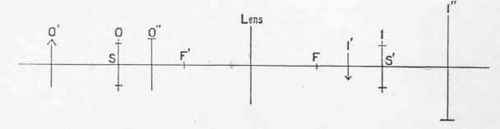Fig.17.To express these relations numerically we must introduce an optical law, known as the "Law of Conjugate Foci" which may be deduced from the geometrical construction above.

If we plot cf and cf' as before, calling c F and cf' each = f and calling the distance between f' and the object o, equal to x, and the

. distance between f' and the image 1 equal to y; the law tells us that f2=xy . . . . (1) which means that the focal length of the lens squared, or multiplied by itself, is always equal to the distance between the object and the front focal point, multiplied by the distance between the image and the back focal point. As this generalisation may at first seem a little confusing to the reader, we will interpret the law in another fashion.

Plot c, f', and f as before, measure the distance between the object o and the front focal point f', and find what multiple this distance is of the focal length of the lens (in the figure it is five times); the distance between the back focal point f and the image 1 will be the reciprocal of this multiple of the focal length (in the figure 1/5 of the focal length), o and 1 are said to be conjugate points, or conjugate to one another. If the object be placed at i the image will be formed at O; so that object and image are interchangeable. The size (linear) of the image will also be one-fifth of the size of the object; in other words, the magnification will be one-fifth.Plate III

Taken with the same 8 1/4" c. de v. lens combined with a 4-in. negative lens, as Plate II., at a distance of 15 ft. {By the Author.)To take a numerical example:

Supposing the focal length of the lens f =10 inches ; then the distance o f' is 5 x 10, or 50 inches ; the distance of the object from the centre of the lens is 60 inches ; the distance 1 f is 10/5 or 2 inches, and the distance of the image from the centre of the lens is 12 inches. The size (linear) of the image is to that of the object as their respectives distances from the centre of the lens, or as 12 : 60, that is as 1: 15; the magnification is one-fifth.

Fig.18.If we substitute the values for the focal length of the lens, the distance from the front focal point to the object, and the distance of the back focal point to the image in the general formula expressing the "law of conjugate foci," we shall find that it holds good.

f2 = xy

10 x 10 = 50x 2 100= 100

In general, then, we notice that the further a given object is from the lens, the smaller will be its image, and the more closely will the image approach to the focal point or plane on the further side of the lens; when the object is very distant, or at "infinity" as it is termed, the image lies in that focal plane.

Conversely, when the object is brought nearer the lens, the plane of the image recedes from the second focal point and increases in size (although the image is smaller than the object), until it arrives at a distance equal to one focal length beyond the front focal point, or, in other words, when it is twice the focal length of the lens away from the centre of the lens. In this interesting position, image and object are of the same size, and are at equal distances from the centre of the lens.

If f be the focal length of the lens, c its centre, f' and f the focal points, and o f' be made =f, it follows that F 1 must =f; for o f is now the multiple one of the focal length, so that F 1 must be the reciprocal of this multiple, that is to say, one also. Or, from the formula : f2 =xy fxf=fxf

Fig. 19.These particular positions of o and 1 are called the symmetric points, and the planes passing through them the symmetric planes; they possess, as we have seen, the property that any object situated in one will be reproduced (but inverted) exactly the same size in image in the other. This is called the position of "unit magnification."

The position of the symmetric points s s' is also remarkable in this respect. If the object is situated beyond one of these on one side of the lens its image is diminished in size (magnification less than unity), and is nearer to the lens than the other: if, on the other hand, the object is situated anywhere between one of them and the focal point on that side of the lens, its image is increased in size (magnification greater than unity), and is further from the lens than the other.

Thus, o lying in s is exactly reproduced at i in s'; o' to the left of s is diminished in size at I', and o" to the right of o, between s and f' is increased in size at I". (Note the interchangeability of object and image in the figure.)

If the object is brought so near to the lens as to coincide with the focal point (or plane through F') we know that, as this point is the position of the focus for parallel rays, the image must be formed at "infinity." Again, if the object be moved nearer still to the lens than this, every ray after passing through the lens will diverge and no real image will be formed. (A virtual image is formed in this case, but it will not be necessary to enter here into the formation of virtual images by positive lenses.)

Fig.20.